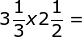# Multiplying Compound Fractions

Instructions:

question 1 of 3

### What is the first step in multiplying compound fractions?

Create Your Account To Take This Quiz

As a member, you'll also get unlimited access to over 79,000 lessons in math, English, science, history, and more. Plus, get practice tests, quizzes, and personalized coaching to help you succeed.

Try it risk-free for 30 days. Cancel anytime

### 2. Solve:Create your account to access this entire worksheet
Quizzes, practice exams & worksheets
Certificate of Completion
Create an account to get started

This quiz/worksheet is made up of questions on core aspects of multiplying compound fractions. You'll be tested on the process of this mathematical operation as well as related sample problems.

## Quiz & Worksheet Goals

This quiz includes questions addressing the following:

• The initial step of multiplying compound fractions
• What is and isn't a step of multiplying compound fractions
• Solving example problems by multiplying compound fractions

## Skills Practiced

• Problem solving - use acquired knowledge to solve practice problems involving the multiplication of compound fractions
• Distinguishing differences - ensure that you can differentiate between steps that do and do not apply to multiplying compound fractions
• Information recall - access the knowledge you've gained regarding the first step of solving compound fractions

To extend your learning of this mathematic process, you can review the corresponding lesson called Multiplying Compound Fractions. This lesson can help you reach the following objectives:

• Define compound fraction
• Describe an improper fraction
• Review provided examples of how to multiply compound fractions
Final ExamMath for Kids
Status: Not Started
Chapter ExamFractions for Elementary School
Status: Not Started

Support